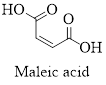Chapter 23, Problem 121SCQ

Chapter
Section
Textbook Problem

Maleic acid is prepared by the catalytic oxidation of benzene. It is a dicarboxylic acid; that is, it has two carboxylic acid groups.(a) Combustion of 0.125 g of the acid gives 0.190 g of CO2 and 0.0388 g of H2O. Calculate the empirical formula of the acid.(b) A 0.261-g sample of the acid requires 34.60 mL of 0.130 M NaOH for complete titration (so that the H ions from both carboxylic acid groups are used). What is the molecular formula of the acid?(c) Draw a Lewis structure for the acid.(d) Describe the hybridization used by the C atoms.(e) What are the bond angles around each C atom?

(a)

Interpretation Introduction

Interpretation:

The empirical formula of the given acid has to be determined.

Concept introduction:

Maleic acid is prepared by the catalytic oxidation of benzene.It is a dicarboxylic acid ,that is, it has two carboxylic acid groups.

It is cis– isomer of butenedioic acid.Molecular formula is C4H4O4.

The structure of maleic acid is as shown below.Empirical formula of a compound is the smallest integer ratio of numbers of each element presented in that compound.

Molecular formula of a compound is integer multiple of empirical formula, the integer is depend upon the mass of empirical formula and the molecular mass of the compound.

The number of moles of any substance can be determined using the equation,

Numberofmole=GivenmassofthesubstanceMolarmass

Explanation

Assuming the molecular formula of the compound is CxHyOz.

It dissociates into carbondioxide and water. The equation is as follows.

CxHyOz CO2+H2O

Let’s calculate the moles of C in CO2:

Moles of C =Given weight of CO2Molecular weight of CO21×0.19044= 4.32×10-3

Weigh of Carbon = 4.32 × 10-3 × 12= 0.0518 g

Let’s calculate the moles of H in H2O.

Moles of H =Given weight of H2OMolecular weight of H2O2×0.038818= 4

(b)

Interpretation Introduction

Interpretation:

The molecular formula of the given acid has to be determined.

Concept introduction:

Maleic acid is prepared by the catalytic oxidation of benzene.It is a dicarboxylic acid ,that is, it has two carboxylic acid groups.

It is cis– isomer of butenedioic acid.Molecular formula is C4H4O4.

The structure of maleic acid is as shown below.Empirical formula of a compound is the smallest integer ratio of numbers of each element presented in that compound.

Molecular formula of a compound is integer multiple of empirical formula, the integer is depend upon the mass of empirical formula and the molecular mass of the compound.

The number of moles of any substance can be determined using the equation,

Numberofmole=GivenmassofthesubstanceMolarmass

(c)

Interpretation Introduction

Interpretation:

The Lewis structure of the given acid has to be drawn.

Concept introduction:

Maleic acid is prepared by the catalytic oxidation of benzene.It is a dicarboxylic acid ,that is, it has two carboxylic acid groups.

It is cis– isomer of butenedioic acid.Molecular formula is C4H4O4.

The structure of maleic acid is as shown below.Lewis structures are diagrams that represent the chemical bonding of covalently bonded molecules and coordination compounds.

It is also known as Lewis dot structures which represent the bonding between atoms of a molecule and the lone pairs of electrons that may exist in the molecule.

(d)

Interpretation Introduction

Interpretation:

The hybridization used by the carbon atom in the given acid compound has to be described.

Concept introduction:

Maleic acid is prepared by the catalytic oxidation of benzene.It is a dicarboxylic acid ,that is, it has two carboxylic acid groups.

It is cis– isomer of butenedioic acid.Molecular formula is C4H4O4.

The structure of maleic acid is as shown below.Hybridization is the mixing of valence atomic orbitals to get equivalent hybridized orbitals that having similar characteristics and energy.

TypeofmoleculeHybridaizationAtomicorbitalsusedforhybridaizationGeometryAX2sp1s+1pLinearAX3,AX2Bsp21s+2pTrigonalplanarAX4,AX3B,AX2B2sp31s+3pTetrahedralAX5,AX4B,AX3B2,AX2B3sp3d1s+3p+1dTrigonalbipyramidalAX6,AX5B,AX4B2sp3d21s+3p+2dOctahedralACentralatomXAtomsbondedtoABNonbondingelectronpairsonA

(e)

Interpretation Introduction

Interpretation:

The bond angles around each C-atom in the given molecule has to be identified.

Concept introduction:

Maleic acid is prepared by the catalytic oxidation of benzene.It is a dicarboxylic acid ,that is, it has two carboxylic acid groups.

It is cis– isomer of butenedioic acid.Molecular formula is C4H4O4.

The structure of maleic acid is as shown below.Hybridization is the mixing of valence atomic orbitals to get equivalent hybridized orbitals that having similar characteristics and energy.

TypeofmoleculeHybridaizationGeometryBondangleAX2spLinear180°AX3,AX2Bsp2Trigonalplanar120°AX4,AX3B,AX2B2sp3Tetrahedral109.5°AX5,AX4B,AX3B2,AX2B3sp3dTrigonalbipyramidal120°,90°AX6,AX5B,AX4B2sp3d2Octahedral90°ACentralatomXAtomsbondedtoABNonbondingelectronpairsonA

Still sussing out bartleby?

Check out a sample textbook solution.

See a sample solution

The Solution to Your Study Problems

Bartleby provides explanations to thousands of textbook problems written by our experts, many with advanced degrees!

Get Started

Find more solutions based on key concepts